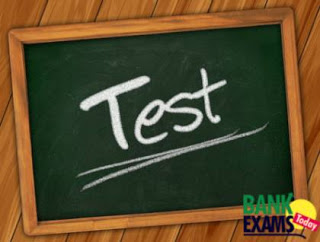# Mixed Aptitude Quiz - 15 ( For SBI Clerical 2016 )#### Ques 1.

3200 sweets were to be equally distributed among certain children equally. But on that day 80 children were absent. Hence each child got 2 sweet extra. How many children were originally there?

Solution :

3200/(x-80) - 3200/x  = 2

==>X = 400

#### Ques 2.

A trader sold an article at 12% loss. Had he sold the same article for Rs. 76.50 more, he would have earned a profit of 5%. What would be the selling price of the article if it is sold at 16% profit ?

Solution :

case-1
cp = 100x sp = 88x loss=12%

case-2
cp=100x sp= 88x+76.50 profit =5%

from case-2

x=4.5

cp =100x =450

450------100%
?      ------116%

==>X = 522

#### Ques 3.

If the lengths of the three sides of a triangle are 6 cm, 8 cm and 10 cm, then the length of the median to its greatest side is
Solution :

8*6/10= 4.8

#### Ques 4.

A, B and C are employed to do a piece of work for Rs.529. A and B together are supposed to do 19/23 of the work and B and C together 8/23 of the work. What amount should A be paid?

Solution :

Total work = 23

B and  C did work= 8

So remaining = 15 by A

A share =529 * 15/23 = 345

#### Ques 5.

a sum of rs.4300 has been distributed among 45 people consisting of Man,Women,and Children.the amount given to man,women and children are in the ratio 12:15:16, but the amount received by each man,woman and Children are in the ratio 6:5:4,what each man,women and child received?

Solution :

43x------4300
X----------100

men=1200
women=1500
children=1600

1200/6x : 1500/5x :1600/4x = 2:3:4

9x----45
X------5

==>10:15:20

Each man=1200/10=120
woman=1500/15=100
children=1600/20=80

#### Ques 6.

The ratio between the number of passengers travelling by 1st and 2nd class between the two railway stations is 1 : 50, whereas the ratio of 1st and 2nd class fares between the same stations is 3 : 1. If an a particular day, Rs. 1325 were collected from the passengers travelling between these stations, then what was the amount collected from the 2nd class passengers?

Solution :

1x:50x

3x:1x

(3x*1x)+(50x*1x) = 1325

x = 25

2nd Class passengers = 50X= 50*25=1250

#### Ques 7.

a/3=b/4=c/7 then find (a+b+c)/c.

Solution :

a:b = 3:4
b:c = 4:7

a:b:c = 3:4:7

(a+b+c)/c = (3+4+7)/7 = 14/7=2

#### Ques 8.

The square of a number X is 80 more than the square of another number Y. If the value of X is1.5 times the value of Y, what is the difference between the value of X and that of Y ?

Solution:

2.25y^2 - y^2 = 80
1.25y^2 = 80
Y = 8
X = 1.5*8 = 12

X - Y = 12 - 8 = 4

#### Ques 9.

If the average of 4 consecutive even numbers is 57. what is the largest number ?

Solution :

4X + 12 = 228
X =54

largest number = 54+6= 60

#### Ques 10.

In college , 65% of the total numbers of students are males and the number of females is 525.What is the total number of males in college ?

Solution :

100-65= 35%

35% ----- 525
65% ----- X

male = X = 975

#### Ques 11.

64  32  48 120  ?   1890

Solution :

64*0.5 = 32
32*1.5 = 48
48*2.5 = 120
120*3.5=420